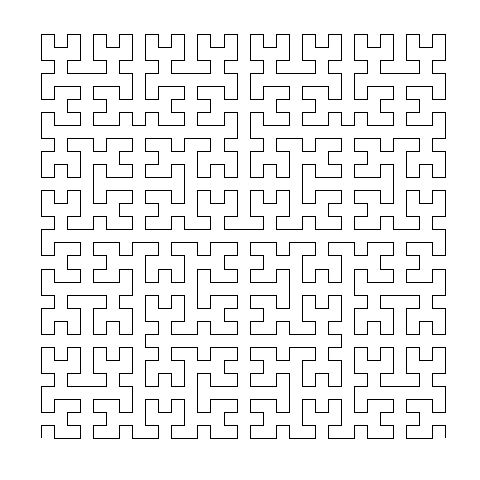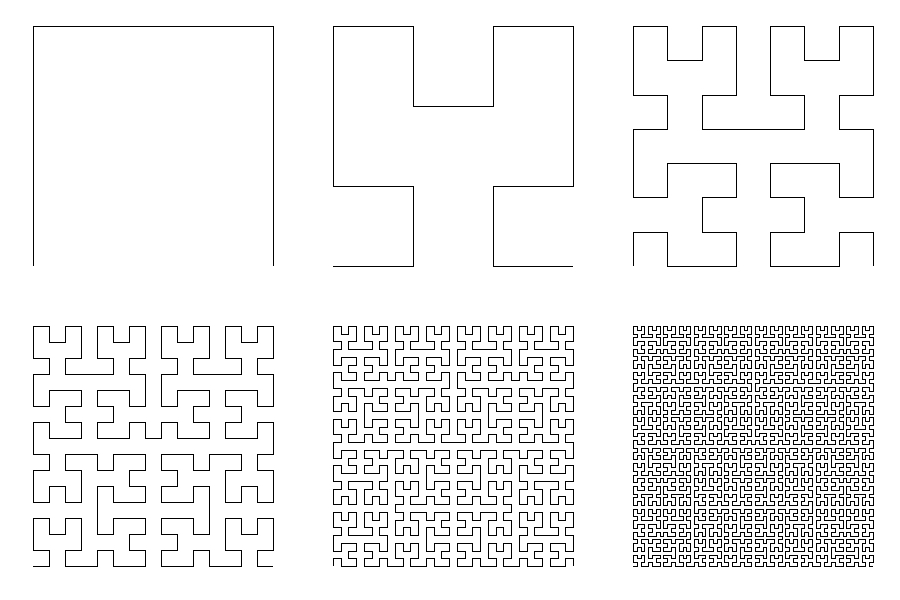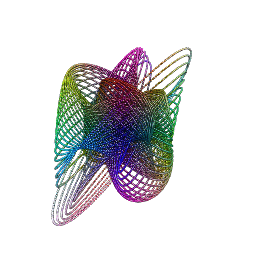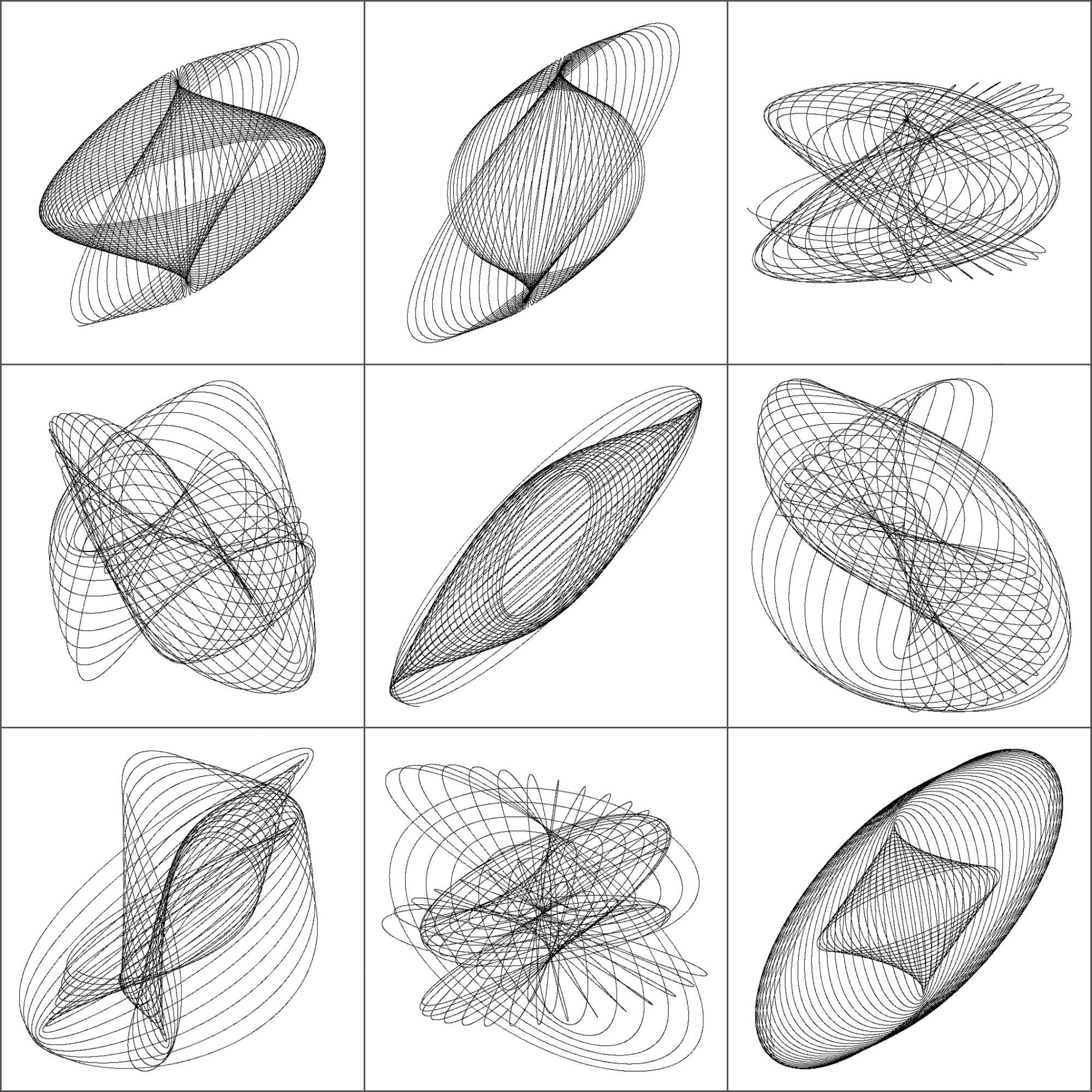# A Shiny App to Draw Curves Based on L-System

Don’t worry about a thing ’cause every little thing gonna be alright (Three Little Birds, Bob Marley)

One of my favourite books is The Computational Beauty of Nature by Gary William Flake where there is a fantastic chapter about fractals in which I discovered the L-Systems.

L-Systems were conceived  in 1968 by Aristide Lindenmayer, a Hungarian biologist, as a mathematical description of plant growth. Apart from the Wikipedia, there are many places on the Internet where you can read about them. If you are interested, don’t miss The Algorithmic Beauty of Plants, an awesome book by Przemysław Prusinkiewicz that you can obtain here for free.

Roughly speaking, a L-System is a very efficient way to make drawings. In its simplest way consists in two different actions: draw a straigh line and change the angle. This is just what you need, for example, to draw a square: draw a straigh line of  any length, turn 90 degrees (without drawing), draw another straigh line of the same length, turn 90 degrees in the same direction, draw, turn and draw again. Denoting `F` as the action of drawing a line of length d and `+` as turning 90 degrees right, the whole process to draw a square can be represented as `F+F+F+F`.

L-Systems are quite simple to program in R. You only need to substitute the rules iteratively into the axiom (I use `gsubfn` function to do it) and split the resulting chain into parts with `str_extract_all`, for example. The result is a set of very simple actions (draw or turn) that can be visualized with `ggplot` and its path geometry. There are four important parameters in L-Systems:

• The seed of the drawing, called axiom
• The substitutions to be applied iteratively, called rules
• How many times to apply substitutions, called depth
• Angle of each turning

For example, let’s define the next L-System:

• Axiom: `F-F-F-F`
• Rule: `F → F−F+F+FF−F−F+F`

The rule means that every `F` must be replaced by `F−F+F+FF−F−F+F` while `+` means right turning and `-` left one. After one iteration, the axiom is replaced by `F-F+F+FF-F-F+F-F-F+F+FF-F-F+F-F-F+F+FF-F-F+F-F-F+F+FF-F-F+F` and iterating again, the new string is `F-F+F+FF-F-F+F-F-F+F+FF-F-F+F+F-F+F+FF-F-F+F+F-F+F+FF-F-F+FF-F+F+FF-F-F+F-F-F+F+FF-F-F+F-F-F+F+FF-F-F+F+F-F+F+FF-F-F+F-F-F+F+FF-F-F+F-F-F+F+FF-F-F+F+F-F+F+FF-F-F+F+F-F+F+FF-F-F+FF-F+F+FF-F-F+F-F-F+F+FF-F-F+F-F-F+F+FF-F-F+F+F-F+F+FF-F-F+F-F-F+F+FF-F-F+F-F-F+F+FF-F-F+F+F-F+F+FF-F-F+F+F-F+F+FF-F-F+FF-F+F+FF-F-F+F-F-F+F+FF-F-F+F-F-F+F+FF-F-F+F+F-F+F+FF-F-F+F-F-F+F+FF-F-F+F-F-F+F+FF-F-F+F+F-F+F+FF-F-F+F+F-F+F+FF-F-F+FF-F+F+FF-F-F+F-F-F+F+FF-F-F+F-F-F+F+FF-F-F+F+F-F+F+FF-F-F+F`. As you can see, the length of the string grows exponentially. Converting last string into actions, produces this drawing, called Koch Island:It is funny how different axioms and rules produce very different drawings. I have done a Shiny App to play with L-systems. Although it is quite simple, it has two interesting features I would like to undeline:

• Delay reactions with `eventReactive` to allow to set depth and angle values before refreshing the plot
• Build a dynamic UI that reacts to user input depending on the curve choosen

There are twelve curves in the application: Koch Island (and 6 variations), cuadratic snowflake, Sierpinsky triangle, hexagonal Gosper, quadratic Gosper and Dragon curve. These are their plots:

The definition of all these curves (axiom and rules) can be found in the first chapter of the Prusinkiewicz’s book. The magic comes when you modify angles and colors. These are some examples among the infinite number of possibilities that can be created:

I enjoyed a lot doing and playing with the app. You can try it here. If you do a nice drawing, please let me know in Twitter or dropping me an email. This is the code of the App:

`ui.R`:

```library(shiny)

shinyUI(fluidPage(
titlePanel("Curves based on L-systems"),

sidebarLayout(
sidebarPanel(
selectInput("cur", "Choose a curve:",
c("","Koch Island",
"Koch Variation 1",
"Koch Variation 2",
"Koch Variation 3",
"Koch Variation 4",
"Koch Variation 5",
"Koch Variation 6",
"Sierpinsky Triangle",
"Dragon Curve",
"Hexagonal Gosper Curve",
selected = ""),

conditionalPanel(
condition = "input.cur != \"\"",
uiOutput("Iterations")),

conditionalPanel(
condition = "input.cur != \"\"",
uiOutput("Angle")),

conditionalPanel(
condition = "input.cur != \"\"",
selectInput("lic", label = "Line color:", choices = colors(), selected = "black")),

conditionalPanel(
condition = "input.cur != \"\"",
selectInput("bac", label = "Background color:", choices = colors(), selected = "white")),

conditionalPanel(
condition = "input.cur != \"\"",
actionButton(inputId = "go", label = "Go!",
style="color: #fff; background-color: #337ab7; border-color: #2e6da4"))

),
mainPanel(plotOutput("curve", height="550px", width = "100%"))
)

))
```

`server.R`:

```library(shiny)
library(gsubfn)
library(stringr)
library(dplyr)
library(ggplot2)
library(rlist)

shinyServer(function(input, output) {

curves=list(
list(name="Koch Island",
axiom="F-F-F-F",
rules=list("F"="F-F+F+FF-F-F+F"),
angle=90,
n=2,
alfa0=90),
axiom="-F",
rules=list("F"="F+F-F-F+F"),
angle=90,
n=4,
alfa0=90),
list(name="Koch Variation 1",
axiom="F-F-F-F",
rules=list("F"="FF-F-F-F-F-F+F"),
angle=90,
n=3,
alfa0=90),
list(name="Koch Variation 2",
axiom="F-F-F-F",
rules=list("F"="FF-F-F-F-FF"),
angle=90,
n=4,
alfa0=90),
list(name="Koch Variation 3",
axiom="F-F-F-F",
rules=list("F"="FF-F+F-F-FF"),
angle=90,
n=3,
alfa0=90),
list(name="Koch Variation 4",
axiom="F-F-F-F",
rules=list("F"="FF-F--F-F"),
angle=90,
n=4,
alfa0=90),
list(name="Koch Variation 5",
axiom="F-F-F-F",
rules=list("F"="F-FF--F-F"),
angle=90,
n=5,
alfa0=90),
list(name="Koch Variation 6",
axiom="F-F-F-F",
rules=list("F"="F-F+F-F-F"),
angle=90,
n=4,
alfa0=90),
list(name="Sierpinsky Triangle",
axiom="R",
rules=list("L"="R+L+R", "R"="L-R-L"),
angle=60,
n=6,
alfa0=0),
list(name="Dragon Curve",
axiom="L",
rules=list("L"="L+R+", "R"="-L-R"),
angle=90,
n=10,
alfa0=90),
list(name="Hexagonal Gosper Curve",
axiom="L",
rules=list("L"="L+R++R-L--LL-R+", "R"="-L+RR++R+L--L-R"),
angle=60,
n=4,
alfa0=60),
axiom="-R",
rules=list("L"="LL-R-R+L+L-R-RL+R+LLR-L+R+LL+R-LR-R-L+L+RR-",
"R"="+LL-R-R+L+LR+L-RR-L-R+LRR-L-RL+L+R-R-L+L+RR"),
angle=90,
n=2,
alfa0=90))

output\$Iterations <- renderUI({ if (input\$cur!="") curve=list.filter(curves, name==input\$cur) else curve=list.filter(curves, name=="Koch Island") iterations=list.select(curve, n) %>% unlist
numericInput("ite", "Depth:", iterations, min = 1, max = (iterations+2))
})

output\$Angle <- renderUI({ curve=list.filter(curves, name==input\$cur) angle=list.select(curve, angle) %>% unlist
numericInput("ang", "Angle:", angle, min = 0, max = 360)
})

data <- eventReactive(input\$go, { curve=list.filter(curves, name==input\$cur) axiom=list.select(curve, axiom) %>% unlist
rules=list.select(curve, rules)[]\$rules
alfa0=list.select(curve, alfa0) %>% unlist

for (i in 1:input\$ite) axiom=gsubfn(".", rules, axiom)
actions=str_extract_all(axiom, "\\d*\\+|\\d*\\-|F|L|R|\\[|\\]|\\|") %>% unlist

points=data.frame(x=0, y=0, alfa=alfa0)
for (i in 1:length(actions))
{
if (actions[i]=="F"|actions[i]=="L"|actions[i]=="R")
{
x=points[nrow(points), "x"]+cos(points[nrow(points), "alfa"]*(pi/180))
y=points[nrow(points), "y"]+sin(points[nrow(points), "alfa"]*(pi/180))
alfa=points[nrow(points), "alfa"]
points %>% rbind(data.frame(x=x, y=y, alfa=alfa)) -> points
}
else{
alfa=points[nrow(points), "alfa"]
points[nrow(points), "alfa"]=eval(parse(text=paste0("alfa",actions[i], input\$ang)))
}
}
return(points)
})

output\$curve <- renderPlot({
ggplot(data(), aes(x, y)) +
geom_path(color=input\$lic) +
coord_fixed(ratio = 1) +
theme(legend.position="none",
panel.background = element_rect(fill=input\$bac),
panel.grid=element_blank(),
axis.ticks=element_blank(),
axis.title=element_blank(),
axis.text=element_blank())
})

})
```

# Gummy Worms

Just keep swimming (Dory in Finding Nemo)

Inspired by this post, I decided to create gummy worms like this:Or these:

When I was young I used to eat them.

Do you want to try? This is the code:

```library(rgl)
library(RColorBrewer)
t=seq(1, 6, by=.04)
f = function(a, b, c, d, e, f, t) exp(-a*t)*sin(t*b+c)+exp(-d*t)*sin(t*e+f)
v1=runif(6,0,1e-02)
v2=runif(6, 2, 3)
v3=runif(6,-pi/2,pi/2)
open3d()
spheres3d(x=f(v1, v2, v3, v1, v2, v3, t),
y=f(v1, v2, v3, v1, v2, v3, t),
z=f(v1, v2, v3, v1, v2, v3, t),
```

# The Coaster Maker by Shiny

The word you invented is well formed and could be used in the Italian language (The Accademia della Crusca regarding to the word “Petaloso”, recently invented by an eight-year-old boy)

Are you tired of your old coasters? Do you like to make things by your own? Do you have a PC and a printer at home? If you answered yes to all these questions, just follow these simple instructions:

• Install R and RStudio in your PC
• Open RStudio and create a new Shiny Web App multiple file (ui.R/server.R)
• Substitute sample code of each file by the code below
• Press Run App
• Press buttom Get your coaster! until you obtain a image you like
• Print the image
• Cut out the image
• Place on the coaster your favorite drinking

These are some examples:

This is the code of ui.R

```#
# This is the user-interface definition of a Shiny web application. You can
# run the application by clicking 'Run App' above.
#
# Find out more about building applications with Shiny here:
#
#    http://shiny.rstudio.com/
#
library(shiny)
shinyUI(fluidPage(
titlePanel("The coaster maker"),
sidebarLayout(
sidebarPanel(
#helpText(),

# adding the new div tag to the sidebar
tags\$p("This coasters are generated by hypocycloid curves.The curve is formed by the locus of a point,
attached to a circle, that rolls on the inside of another circle.
In the curve's equation the first part denotes the relative position between the two circles,
the second part denotes the rotation of the rolling circle.")),
HTML(
),
mainPanel(
plotOutput("HarmPlot")
)
)
))
```

This is the code of server.R

```# This is the server logic of a Shiny web application. You can run the
# application by clicking 'Run App' above.
#
# Find out more about building applications with Shiny here:
#
#    http://shiny.rstudio.com/
#
library(shiny)
library(ggplot2)
CreateDS = function ()
{
t=seq(-31*pi, 31*pi, 0.002)
a=sample(seq(from=1/31, to=29/31, by=2/31), 1)
b=runif(1, min = 1, max = 3)
data.frame(x=(1-a)*cos(a*t)+a*b*cos((1-a)*t), y=(1-a)*sin(a*t)-a*b*sin((1-a)*t))
}
shinyServer(function(input, output) {
dat<-reactive({if (input\$rerun) dat=CreateDS() else dat=CreateDS()})
output\$HarmPlot<-renderPlot({
ggplot(dat())+
geom_point(data=data.frame(x=0,y=0), aes(x,y), color=rgb(rbeta(1, .5, .5), rbeta(1, .5, .5), rbeta(1, .5, .5)) , shape=19, fill="yellow", size=220)+
geom_polygon(aes(x, y), fill=rgb(rbeta(1, 2, 2), rbeta(1, 2, 2), rbeta(1, 2, 2))) +
theme(legend.position="none",
panel.background = element_rect(fill="white"),
panel.grid=element_blank(),
axis.ticks=element_blank(),
axis.title=element_blank(),
axis.text=element_blank())
}, height = 500, width = 500)
})
```

# Going Bananas With Hilbert

It seemed that everything is in ruins, and that all the basic mathematical concepts have lost their meaning (Naum Vilenkin, Russian mathematician, regarding to the discovery of Peano’s curve)

Giuseppe Peano found in 1890 a way to draw a curve in the plane that filled the entire space: just a simple line covering completely a two dimensional plane. Its discovery meant a big earthquake in the traditional structure of mathematics. Peano’s curve was the first but not the last: one of these space-filling curves was discovered by Hilbert and takes his name. It is really beautiful:Hilbert’s curve can be created iteratively. These are the first six iterations of its construction:As you will see below, R code to create Hilbert’s curve is extremely easy. It is also very easy to play with the curve, altering the order in which points are sorted. Changing the initial `matrix(1)` by some other number, resulting curves are quite appealing:

Let’s go futher. Changing `ggplot` geometry from `geom_path` to `geom_polygon` generate some crazy pseudo-tessellations:

And what if you change the matrix exponent?

And what if you apply polar coordinates?

We started with a simple line and with some small changes we have created fantastical images. And all these things only using black and white. Do you want to add some colors? Try with the following code (if you draw something interesting, please let me know):

```library(reshape2)
library(dplyr)
library(ggplot2)
opt=theme(legend.position="none",
panel.background = element_rect(fill="white"),
panel.grid=element_blank(),
axis.ticks=element_blank(),
axis.title=element_blank(),
axis.text=element_blank())
hilbert = function(m,n,r) {
for (i in 1:n)
{
tmp=cbind(t(m), m+nrow(m)^2)
m=rbind(tmp, (2*nrow(m))^r-tmp[nrow(m):1,]+1)
}
melt(m) %>% plyr::rename(c("Var1" = "x", "Var2" = "y", "value"="order")) %>% arrange(order)}
# Original
ggplot(hilbert(m=matrix(1), n=1, r=2), aes(x, y)) + geom_path()+ opt
ggplot(hilbert(m=matrix(1), n=2, r=2), aes(x, y)) + geom_path()+ opt
ggplot(hilbert(m=matrix(1), n=3, r=2), aes(x, y)) + geom_path()+ opt
ggplot(hilbert(m=matrix(1), n=4, r=2), aes(x, y)) + geom_path()+ opt
ggplot(hilbert(m=matrix(1), n=5, r=2), aes(x, y)) + geom_path()+ opt
ggplot(hilbert(m=matrix(1), n=6, r=2), aes(x, y)) + geom_path()+ opt
# Changing order
ggplot(hilbert(m=matrix(.5), n=5, r=2), aes(x, y)) + geom_path()+ opt
ggplot(hilbert(m=matrix(0), n=5, r=2), aes(x, y)) + geom_path()+ opt
ggplot(hilbert(m=matrix(tan(1)), n=5, r=2), aes(x, y)) + geom_path()+ opt
ggplot(hilbert(m=matrix(3), n=5, r=2), aes(x, y)) + geom_path()+ opt
ggplot(hilbert(m=matrix(-1), n=5, r=2), aes(x, y)) + geom_path()+ opt
ggplot(hilbert(m=matrix(log(.1)), n=5, r=2), aes(x, y)) + geom_path()+ opt
ggplot(hilbert(m=matrix(-15), n=5, r=2), aes(x, y)) + geom_path()+ opt
ggplot(hilbert(m=matrix(-0.001), n=5, r=2), aes(x, y)) + geom_path()+ opt
# Polygons
ggplot(hilbert(m=matrix(log(1)), n=4, r=2), aes(x, y)) + geom_polygon()+ opt
ggplot(hilbert(m=matrix(.5), n=4, r=2), aes(x, y)) + geom_polygon()+ opt
ggplot(hilbert(m=matrix(tan(1)), n=5, r=2), aes(x, y)) + geom_polygon()+ opt
ggplot(hilbert(m=matrix(-15), n=4, r=2), aes(x, y)) + geom_polygon()+ opt
ggplot(hilbert(m=matrix(-25), n=4, r=2), aes(x, y)) + geom_polygon()+ opt
ggplot(hilbert(m=matrix(0), n=4, r=2), aes(x, y)) + geom_polygon()+ opt
ggplot(hilbert(m=matrix(1000000), n=4, r=2), aes(x, y)) + geom_polygon()+ opt
ggplot(hilbert(m=matrix(-1), n=4, r=2), aes(x, y)) + geom_polygon()+ opt
ggplot(hilbert(m=matrix(-.00001), n=4, r=2), aes(x, y)) + geom_polygon()+ opt
# Changing exponent
gplot(hilbert(m=matrix(log(1)), n=4, r=-1), aes(x, y)) + geom_polygon()+ opt
ggplot(hilbert(m=matrix(.5), n=4, r=-2), aes(x, y)) + geom_polygon()+ opt
ggplot(hilbert(m=matrix(tan(1)), n=4, r=6), aes(x, y)) + geom_polygon()+ opt
ggplot(hilbert(m=matrix(-15), n=3, r=sin(2)), aes(x, y)) + geom_polygon()+ opt
ggplot(hilbert(m=matrix(-25), n=4, r=-.0001), aes(x, y)) + geom_polygon()+ opt
ggplot(hilbert(m=matrix(0), n=4, r=200), aes(x, y)) + geom_polygon()+ opt
ggplot(hilbert(m=matrix(1000000), n=3, r=.5), aes(x, y)) + geom_polygon()+ opt
ggplot(hilbert(m=matrix(-1), n=4, r=sqrt(2)), aes(x, y)) + geom_polygon()+ opt
ggplot(hilbert(m=matrix(-.00001), n=4, r=52), aes(x, y)) + geom_polygon()+ opt
# Polar coordinates
ggplot(hilbert(m=matrix(1), n=4, r=2), aes(x, y)) + geom_polygon()+ coord_polar()+opt
ggplot(hilbert(m=matrix(-1), n=5, r=2), aes(x, y)) + geom_polygon()+ coord_polar()+opt
ggplot(hilbert(m=matrix(.1), n=2, r=.5), aes(x, y)) + geom_polygon()+ coord_polar()+opt
ggplot(hilbert(m=matrix(1000000), n=2, r=.1), aes(x, y)) + geom_polygon()+ coord_polar()+opt
ggplot(hilbert(m=matrix(.25), n=3, r=3), aes(x, y)) + geom_polygon()+ coord_polar()+opt
ggplot(hilbert(m=matrix(tan(1)), n=5, r=1), aes(x, y)) + geom_polygon()+ coord_polar()+opt
ggplot(hilbert(m=matrix(1), n=4, r=1), aes(x, y)) + geom_polygon()+ coord_polar()+opt
ggplot(hilbert(m=matrix(log(1)), n=3, r=sin(2)), aes(x, y)) + geom_polygon()+ coord_polar()+opt
ggplot(hilbert(m=matrix(-.0001), n=4, r=25), aes(x, y)) + geom_polygon()+ coord_polar()+opt
```

# The 2D-Harmonograph In Shiny

If you wish to make an apple pie from scratch, you must first invent the universe (Carl Sagan)

I like Shiny and I can’t stop converting into apps some of my previous experiments: today is the turn of the harmonograph. This is a simple application since you only can press a button to generate a random harmonograph-simulated curve. I love how easy is to create a nice interactive app to play with from an existing code. The only trick in this case in to add a `rerun` button in the UI side and transfer the interaction to the server side using a simple `if`. Very easy. This is a screenshot of the application:Press the button and you will get a new drawing. Most of them are nice scrawls and from time to time you will obtain beautiful shapely curves.

And no more candy words: It is time to complain. I say to RStudio with all due respect, you are very cruel. You let me to deploy my previous app to your server but you suspended it almost immediately for fifteen days due to “exceeded usage hours”. My only option is paying at least \$440 per year to upgrade my current plan. I tried the ambrosia for an extremely short time. RStudio: Why don’t you launch a cheaper account? Why don’t you launch a free account with just one perpetual alive app at a time? Why don’t you increase the usage hours threshold? I can help you to calculate the return on investment of these scenarios.

Or, Why don’t you make me a gift for my next birthday? I promise to upload a new app per month to promote your stunning tool. Think about it and please let me know your conclusions.

Meanwhile I will run the app privately. This is the code to do it:

UI.R

```# This is the user-interface definition of a Shiny web application.
# You can find out more about building applications with Shiny here:
#
# http://www.rstudio.com/shiny/

library(shiny)

shinyUI(fluidPage(
titlePanel("Mathematical Beauties: The Harmonograph"),
sidebarLayout(
sidebarPanel(
#helpText(),

# adding the new div tag to the sidebar
tags\$p("A harmonograph is a mechanical apparatus that employs pendulums to create a
geometric image. The drawings created typically are Lissajous curves, or
related drawings of greater complexity. The devices, which began to appear
in the mid-19th century and peaked in popularity in the 1890s, cannot be
conclusively attributed to a single person, although Hugh Blackburn, a professor
of mathematics at the University of Glasgow, is commonly believed to be the official
inventor. A simple, so-called \"lateral\" harmonograph uses two pendulums to control the movement
of a pen relative to a drawing surface. One pendulum moves the pen back and forth along
one axis and the other pendulum moves the drawing surface back and forth along a
perpendicular axis. By varying the frequency and phase of the pendulums relative to
one another, different patterns are created. Even a simple harmonograph as described
can create ellipses, spirals, figure eights and other Lissajous figures (Source: Wikipedia)")),
HTML("

Click <a href=\"http://paulbourke.net/geometry/harmonograph/harmonograph3.html\">here</a> to see an image of a real harmonograph

")
),
actionButton('rerun','Launch the harmonograph!')
),
mainPanel(
plotOutput("HarmPlot")
)
)
))
```

server.R

```# This is the server logic for a Shiny web application.
# You can find out more about building applications with Shiny here:
#
# http://www.rstudio.com/shiny/
#

library(shiny)

CreateDS = function ()
{

f=jitter(sample(c(2,3),4, replace = TRUE))
d=runif(4,0,1e-02)
p=runif(4,0,pi)
xt = function(t) exp(-d*t)*sin(t*f+p)+exp(-d*t)*sin(t*f+p)
yt = function(t) exp(-d*t)*sin(t*f+p)+exp(-d*t)*sin(t*f+p)
t=seq(1, 200, by=.0005)
data.frame(t=t, x=xt(t), y=yt(t))}

shinyServer(function(input, output) {
dat<-reactive({if (input\$rerun) dat=CreateDS() else dat=CreateDS()})
output\$HarmPlot<-renderPlot({
plot(rnorm(1000),xlim =c(-2,2), ylim =c(-2,2), type="n")
with(dat(), plot(x,y, type="l", xlim =c(-2,2), ylim =c(-2,2), xlab = "", ylab = "", xaxt='n', yaxt='n', col="gray10", bty="n"))
}, height = 650, width = 650)
})
```

# 3D-Harmonographs In Motion

I would be delighted to co write a post (Andrew Wyer)

One of the best things about writing a blog is that occasionally you get to know very interesting people. Last October 13th I published this post about the harmonograph, a machine driven by pendulums which creates amazing curves. Two days later someone called Andrew Wyer made this comment about the post:

Hi, I was fascinated by the harmonographs – I remember seeing similar things done on paper on kids tv in the seventies. I took your code and extended it into 3d so I could experiment with the rgl package. I created some beautiful figures (which I would attach if this would let me). In lieu of that here is the code:

I ran his code and I was instantly fascinated: resulting curves were really beautiful. I suggested that we co-write a post and he was delighted with the idea. He proposed to me the following improvement of his own code:

I will try to create an animated gif of one figure

Such a good idea! And no sooner said than done: Andrew rewrote his own code to create stunning animated images of 3D-Harmonograph curves like these:• Andrew creates 3D curves by adding a third oscillation z generated in the same way as x and y and adds a little colour by setting the colour of each point to a colour in the RGB scale related to its point in 3d space
• Function `spheres3d `to produce an interactive plot that you can drag around to view from different angles; function `spin3d `will rotate the figure around the z axis and at 5 rpm in this case and function `movie3d `renders each frame in a temporary png file and then calls ImageMagick to stitch them into an animated gif file. It is necessary to install ImageMagick separately to create the movie.
• Giving it a duration of 12 seconds at 5 rpm is one rotation which at 12 frames per second results in 144 individual png files but these (by default) are temporary and deleted when the gif is produced.

Although I don’t know Andrew personally, I know he is a good partner to work with. Thanks a lot for sharing this work of art with me and allowing me to share it in Ripples as well.

Here you have the code. I like to imagine these curves as orbits of unexplored planets in a galaxy far, far away …

```library(rgl)
library(scatterplot3d)
#Extending the harmonograph into 3d
#Antonio's functions creating the oscillations
xt = function(t) exp(-d1*t)*sin(t*f1+p1)+exp(-d2*t)*sin(t*f2+p2)
yt = function(t) exp(-d3*t)*sin(t*f3+p3)+exp(-d4*t)*sin(t*f4+p4)
#Plus one more
zt = function(t) exp(-d5*t)*sin(t*f5+p5)+exp(-d6*t)*sin(t*f6+p6)
#Sequence to plot over
t=seq(1, 100, by=.001)
#generate some random inputs
f1=jitter(sample(c(2,3),1))
f2=jitter(sample(c(2,3),1))
f3=jitter(sample(c(2,3),1))
f4=jitter(sample(c(2,3),1))
f5=jitter(sample(c(2,3),1))
f6=jitter(sample(c(2,3),1))
d1=runif(1,0,1e-02)
d2=runif(1,0,1e-02)
d3=runif(1,0,1e-02)
d4=runif(1,0,1e-02)
d5=runif(1,0,1e-02)
d6=runif(1,0,1e-02)
p1=runif(1,0,pi)
p2=runif(1,0,pi)
p3=runif(1,0,pi)
p4=runif(1,0,pi)
p5=runif(1,0,pi)
p6=runif(1,0,pi)
#and turn them into oscillations
x = xt(t)
y = yt(t)
z = zt(t)
#create values for colours normalised and related to x,y,z coordinates
cr = abs(z)/max(abs(z))
cg = abs(x)/max(abs(x))
cb = abs(y)/max(abs(y))
dat=data.frame(t, x, y, z, cr, cg ,cb)
#plot the black and white version
with(dat, scatterplot3d(x,y,z, pch=16,cex.symbols=0.25, axis=FALSE ))
with(dat, scatterplot3d(x,y,z, pch=16, color=rgb(cr,cg,cb),cex.symbols=0.25, axis=FALSE ))
#Set the stage for 3d plots
# clear scene:
clear3d("all")
# white background
bg3d(color="white")
#lights...camera...
light3d()
#action
# draw shperes in an rgl window
#create animated gif (call to ImageMagic is automatic)
movie3d( spin3d(axis=c(0,0,1),rpm=5),fps=12, duration=12 )
#2d plots to give plan and elevation shots
plot(x,y,col=rgb(cr,cg,cb),cex=.05)
plot(y,z,col=rgb(cr,cg,cb),cex=.05)
plot(x,z,col=rgb(cr,cg,cb),cex=.05)
```

# Beautiful Curves: The Harmonograph

Each of us has their own mappa mundi (Gala, my indispensable friend)

The harmonograph is a mechanism which, by means of several pendulums, draws trajectories that can be analyzed not only from a mathematical point of view but also from an artistic one. In its double pendulum version, one pendulum moves a pencil and the other one moves a platform with a piece of paper on it. You can see an example here. The harmonograph is easy to use: you only have to put pendulums into motion and wait for them to stop. The result are amazing undulating drawings like this one:First harmonographs were built in 1857 by Scottish mathematician Hugh Blackburn, based on the previous work of French mathematician Jean Antoine Lissajous. There is not an unique way to describe mathematically the motion of the pencil. I have implemented the one I found in this sensational blog, where motion in both x and y axis depending on time is defined by:$
x(t)=e^{-d_{1}t}sin(f_{1}t+p_{1})+e^{-d_{2}t}sin(f_{2}t+p_{2})\\
y(t)=e^{-d_{3}t}sin(f_{3}t+p_{3})+e^{-d_{4}t}sin(f_{4}t+p_{4})
$

I initialize parameters randomly so every time you run the script, you obtain a different output. Here is a mosaic with some of mine:This is the code to simulate the harmonograph (no extra package is required). If you create some nice work of art, I will be very happy to admire it (you can find my email here):

```f1=jitter(sample(c(2,3),1));f2=jitter(sample(c(2,3),1));f3=jitter(sample(c(2,3),1));f4=jitter(sample(c(2,3),1))
d1=runif(1,0,1e-02);d2=runif(1,0,1e-02);d3=runif(1,0,1e-02);d4=runif(1,0,1e-02)
p1=runif(1,0,pi);p2=runif(1,0,pi);p3=runif(1,0,pi);p4=runif(1,0,pi)
xt = function(t) exp(-d1*t)*sin(t*f1+p1)+exp(-d2*t)*sin(t*f2+p2)
yt = function(t) exp(-d3*t)*sin(t*f3+p3)+exp(-d4*t)*sin(t*f4+p4)
t=seq(1, 100, by=.001)
dat=data.frame(t=t, x=xt(t), y=yt(t))
with(dat, plot(x,y, type="l", xlim =c(-2,2), ylim =c(-2,2), xlab = "", ylab = "", xaxt='n', yaxt='n'))
```